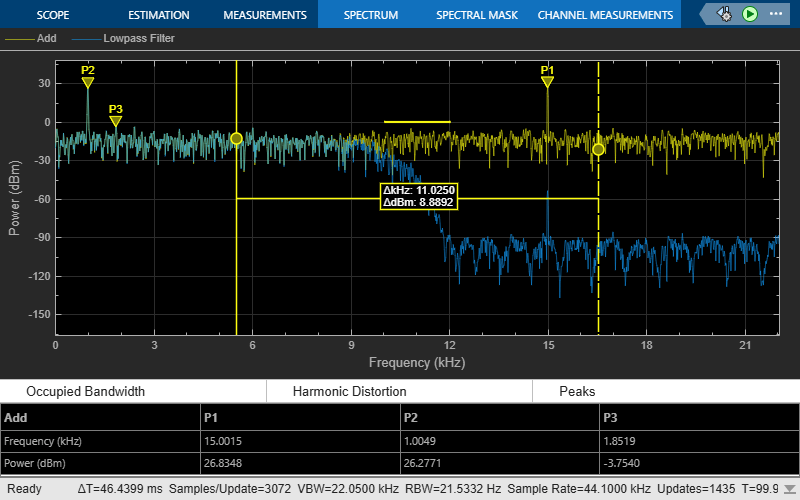# getMeasurementsData

Get the current measurement data displayed on the spectrum analyzer

Since R2018b

## Syntax

``data = getMeasurementsData(scope)``
``data = getMeasurementsData(scope,"all")``

## Description

example

````data = getMeasurementsData(scope)` returns a data table about the current spectrum analyzer measurements in use.```
````data = getMeasurementsData(scope,"all")` returns a data table about all spectrum analyzer measurements for the current time step.```

## Examples

collapse all

Compute and display the power spectrum of a noisy sinusoidal input signal using the `spectrumAnalyzer` MATLAB® object. Measure the peaks, cursor placements, adjacent channel power ratio, and distortion values in the spectrum by enabling these properties:

• `PeakFinder`

• `CursorMeasurements`

• `ChannelMeasurements`

• `DistortionMeasurements`

Initialization

The input sine wave has two frequencies: 1000 Hz and 5000 Hz. Create two `dsp.SineWave` System objects to generate these two frequencies. Create a `spectrumAnalyzer` object to compute and display the power spectrum.

```Fs = 44100; Sineobject1 = dsp.SineWave(SamplesPerFrame=1024,PhaseOffset=10,... SampleRate=Fs,Frequency=1000); Sineobject2 = dsp.SineWave(SamplesPerFrame=1024,... SampleRate=Fs,Frequency=5000); SA = spectrumAnalyzer(SampleRate=Fs,SpectrumType="power",... PlotAsTwoSidedSpectrum=false,ChannelNames={'Power spectrum of the input'},... YLimits=[-120 40],ShowLegend=true);```

Enable Measurements Data

To obtain the measurements, set the `Enabled` property to `true`.

```SA.CursorMeasurements.Enabled = true; SA.ChannelMeasurements.Enabled = true; SA.PeakFinder.Enabled = true; SA.DistortionMeasurements.Enabled = true;```

Use `getMeasurementsData`

Stream in the noisy sine wave input signal and estimate the power spectrum of the signal using the `spectrumAnalyzer` object. Measure the characteristics of the spectrum. Use the `getMeasurementsData` function to obtain these measurements programmatically. The `isNewDataReady` function returns `true` when there is new spectrum data. Store the measured data in the variable `data`.

```data = []; for Iter = 1:1000 Sinewave1 = Sineobject1(); Sinewave2 = Sineobject2(); Input = Sinewave1 + Sinewave2; NoisyInput = Input + 0.001*randn(1024,1); SA(NoisyInput); if SA.isNewDataReady data = [data;getMeasurementsData(SA)]; end end```The panes at the bottom of the scope window display the measurements that you have enabled. The values in these panes match the values in the last time step of the `data` variable. You can access the individual fields of `data` to obtain the various measurements programmatically.

Compare Peak Values

Use the `PeakFinder` property to obtain peak values. Verify that the peak values in the last time step of `data` match the values in the spectrum analyzer plot.

`peakvalues = data.PeakFinder(end).Value `
```peakvalues = 3×1 26.3957 22.7830 -57.9977 ```
`frequencieskHz = data.PeakFinder(end).Frequency/1000`
```frequencieskHz = 3×1 4.9957 0.9905 20.6719 ```

Compute and display the power spectrum of a noisy sinusoidal input signal using the Spectrum Analyzer block. Measure the cursor placements, adjacent channel power ratio, distortion, and peak values in the spectrum by enabling these block configuration properties:

• CursorMeasurements

• ChannelMeasurements

• DistortionMeasurements

• PeakFinder

Open and Inspect the Model

Filter a streaming noisy sinusoidal input signal using a Lowpass Filter block. The input signal consists of two sinusoidal tones: 1 kHz and 15 kHz. The noise is white Gaussian noise with a mean of 0 and a variance of 0.05. The sampling frequency is 44.1 kHz. Open the model and inspect the parameter values in the blocks.

```model = 'spectrumanalyzer_measurements.slx'; open_system(model) ```Access the configuration properties of the Spectrum Analyzer block using the `get_param` function.

```sablock = 'spectrumanalyzer_measurements/Spectrum Analyzer'; cfg = get_param(sablock,'ScopeConfiguration'); ```

Enable Measurements Data

To obtain the measurements, set the `Enabled` property to `true`.

```cfg.CursorMeasurements.Enabled = true; cfg.ChannelMeasurements.Enabled = true; cfg.DistortionMeasurements.Enabled = true; cfg.PeakFinder.Enabled = true; ```

Simulate the Model

Run the model. The Spectrum Analyzer block compares the original spectrum with the filtered spectrum.

```sim(model) ```The panes at the bottom of the spectrum analyzer window display the measurements that you have enabled.

Use `getMeasurementsData` function

Use the `getMeasurementsData` function to obtain the measurements programmatically.

```data = getMeasurementsData(cfg) ```
```data = 1x5 table SimulationTime PeakFinder CursorMeasurements ChannelMeasurements DistortionMeasurements ______________ __________ __________________ ___________________ ______________________ 9.9962 1x1 struct 1x1 struct 1x1 struct 1x1 struct ```

The values shown in the measurement panels match the values shown in `data`. You can access the individual fields of `data` to obtain the various measurements programmatically.

Compare Peak Values

As an example, compare the peak values. Verify that the peak values obtained by `data.PeakFinder` match with the values in the spectrum analyzer window.

```peakvalues = data.PeakFinder.Value frequencieskHz = data.PeakFinder.Frequency/1000 ```
```peakvalues = 26.9642 26.3047 -4.5211 frequencieskHz = 15.0015 1.0049 1.6939 ```

## Input Arguments

collapse all

Spectrum Analyzer you want to query, specified as one of the following:

## Output Arguments

collapse all

Measurements data, returned as a table. When you specify `"all"`, the function returns a measurements table containing the following fields.

FieldDescription
`SimulationTime`Simulation time
`PeakFinder`Peak finder data
`CursorMeasurements`Cursor measurements data
`ChannelMeasurements`Channel measurements data
`DistortionMeasurements`Distortion measurements data
`CCDFMeasurements` (applies only for `SpectrumAnalyzerConfiguration` object)CCDF measurements data

When you do not specify `"all"`, the data table contains only the Spectrum Analyzer measurements currently in use.

## Version History

Introduced in R2018b

expand all